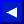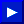The AND operation results in a 1 only if both bits are 1: 1 AND 1 = 1 0 AND 1 = 0 1 AND 0 = 0 0 AND 0 = 0 The OR operation results in a 1 if either bit is 1: 1 OR 1 = 1 0 OR 1 = 1 0 OR 0 = 1 0 OR 0 = 0 The NOT operation logically complements each bit: NOT 1 = 0 NOT 0 = 1 The exclusive OR (XOR) is part of the WAIT statement! 1 XOR 1 = 0 1 XOR 0 = 1 0 XOR 1 = 1 0 XOR 0 = 0

The logical operators AND, OR and NOT specify a Boolean arithmetic operation to be performed on the two operand expressions on either side of the operator. In the case of NOT, ONLY the operand on the RIGHT is considered. Logical operations (or Boolean arithmetic) aren't performed until all arithmetic and relational operations in an expression have been completed.

EXAMPLES:
 ` IF A=100 AND B=100 THEN 10 ` (if both A and B have a value of 100 then the result is true) ` A=96 AND 32: PRINT A ` (A = 32)This page has been created by Sami Rautiainen. Read the small print. Last updated November 13, 1998.